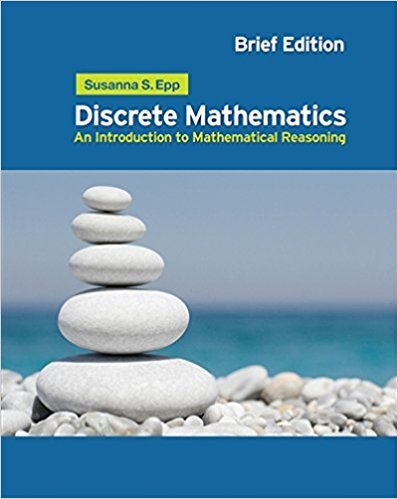×
×

# Solutions for Chapter 7: Functions## Full solutions for Discrete Mathematics: Introduction to Mathematical Reasoning | 1st Edition

ISBN: 9780495826170Solutions for Chapter 7: Functions

Solutions for Chapter 7
4 5 0 349 Reviews
24
1
##### ISBN: 9780495826170

Discrete Mathematics: Introduction to Mathematical Reasoning was written by and is associated to the ISBN: 9780495826170. This textbook survival guide was created for the textbook: Discrete Mathematics: Introduction to Mathematical Reasoning, edition: 1. Since 194 problems in chapter 7: Functions have been answered, more than 16551 students have viewed full step-by-step solutions from this chapter. Chapter 7: Functions includes 194 full step-by-step solutions. This expansive textbook survival guide covers the following chapters and their solutions.

Key Math Terms and definitions covered in this textbook
• Covariance matrix:E.

When random variables Xi have mean = average value = 0, their covariances "'£ ij are the averages of XiX j. With means Xi, the matrix :E = mean of (x - x) (x - x) T is positive (semi)definite; :E is diagonal if the Xi are independent.

• Dimension of vector space

dim(V) = number of vectors in any basis for V.

• Exponential eAt = I + At + (At)2 12! + ...

has derivative AeAt; eAt u(O) solves u' = Au.

• Fast Fourier Transform (FFT).

A factorization of the Fourier matrix Fn into e = log2 n matrices Si times a permutation. Each Si needs only nl2 multiplications, so Fnx and Fn-1c can be computed with ne/2 multiplications. Revolutionary.

• Gauss-Jordan method.

Invert A by row operations on [A I] to reach [I A-I].

• Hankel matrix H.

Constant along each antidiagonal; hij depends on i + j.

• Independent vectors VI, .. " vk.

No combination cl VI + ... + qVk = zero vector unless all ci = O. If the v's are the columns of A, the only solution to Ax = 0 is x = o.

• Inverse matrix A-I.

Square matrix with A-I A = I and AA-l = I. No inverse if det A = 0 and rank(A) < n and Ax = 0 for a nonzero vector x. The inverses of AB and AT are B-1 A-I and (A-I)T. Cofactor formula (A-l)ij = Cji! detA.

• Multiplication Ax

= Xl (column 1) + ... + xn(column n) = combination of columns.

• Orthonormal vectors q 1 , ... , q n·

Dot products are q T q j = 0 if i =1= j and q T q i = 1. The matrix Q with these orthonormal columns has Q T Q = I. If m = n then Q T = Q -1 and q 1 ' ... , q n is an orthonormal basis for Rn : every v = L (v T q j )q j •

• Outer product uv T

= column times row = rank one matrix.

• Positive definite matrix A.

Symmetric matrix with positive eigenvalues and positive pivots. Definition: x T Ax > 0 unless x = O. Then A = LDLT with diag(D» O.

• Projection matrix P onto subspace S.

Projection p = P b is the closest point to b in S, error e = b - Pb is perpendicularto S. p 2 = P = pT, eigenvalues are 1 or 0, eigenvectors are in S or S...L. If columns of A = basis for S then P = A (AT A) -1 AT.

• Projection p = a(aTblaTa) onto the line through a.

P = aaT laTa has rank l.

• Semidefinite matrix A.

(Positive) semidefinite: all x T Ax > 0, all A > 0; A = any RT R.

• Skew-symmetric matrix K.

The transpose is -K, since Kij = -Kji. Eigenvalues are pure imaginary, eigenvectors are orthogonal, eKt is an orthogonal matrix.

• Spectral Theorem A = QAQT.

Real symmetric A has real A'S and orthonormal q's.

• Triangle inequality II u + v II < II u II + II v II.

For matrix norms II A + B II < II A II + II B II·

• Vandermonde matrix V.

V c = b gives coefficients of p(x) = Co + ... + Cn_IXn- 1 with P(Xi) = bi. Vij = (Xi)j-I and det V = product of (Xk - Xi) for k > i.

• Vector v in Rn.

Sequence of n real numbers v = (VI, ... , Vn) = point in Rn.

×